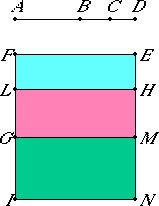# Proposition 84

To a straight line which produces with a medial area a medial whole only one straight line can be annexed which is incommensurable in square with the whole straight line and which with the whole straight line makes the sum of squares on them medial and twice the rectangle contained by them both medial and also incommensurable with the sum of the squares on them.
X.78

Let AB be the straight line which produces with a medial area a medial whole, and BC an annex to it. Then AC and CB are straight lines incommensurable in square which fulfill the aforesaid conditions.

I say that no other straight line can be annexed to AB which fulfills the aforesaid conditions.

X.78

If possible, let BD be so annexed, so that AD and DB are also straight lines incommensurable in square which make the squares on AD and DB added together medial, twice the rectangle AD by DB medial, and also the sum of the squares on AD and DB incommensurable with twice the rectangle AD by DB.II.7

Set out a rational straight line EF. Apply EG, equal to the sum of the squares on AC and CB, to EF producing EM as breadth. Apply HG, equal to twice the rectangle AC by CB, to EF producing HM as breadth. Then the remainder, the square on AB, equals EL. Therefore AB is the side of EL.

Again, apply EI, equal to the sum of the squares on AD and DB, to EF producing EN as breadth.

II.7

But the square on AB also equals EL, therefore the remainder, twice the rectangle AD by DB, equals HI.

X.22

Now, since the sum of the squares on AC and CB is medial and equals EG, therefore EG is also medial. And it is applied to the rational straight line EF producing EM as breadth, therefore EM is rational and incommensurable in length with EF.

X.22

Again, since twice the rectangle AC by CB is medial and equals HG, therefore HG is also medial. And it is applied to the rational straight line EF producing HM as breadth, therefore HM is rational and incommensurable in length with EF.

Since the sum of the squares on AC and CB is incommensurable with twice the rectangle AC by CB, therefore EG is also incommensurable with HG. Therefore EM is also incommensurable in length with MH.

X.73

And both are rational, therefore EM and MH are rational straight lines commensurable in square only. Therefore EH is an apotome, and HM an annex to it.

X.79

Similarly we can prove that EH is again an apotome and HN an annex to it. Therefore to an apotome different rational straight lines are annexed which are commensurable with the wholes in square only, which was proved impossible.

Therefore no other straight line can be so annexed to AB. Therefore to AB only one straight line can be annexed which is incommensurable in square with the whole and which with the whole makes the squares on them added together medial, twice the rectangle contained by them medial, and also the sum of the squares on them incommensurable with twice the rectangle contained by them.

Q.E.D.

## Guide

This proposition is not used in the rest of the Elements.